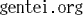ProjectG \$B\$G1?1D\$7\$F\$\$\$k(BML\$B0lMw\$G\$9!#(B
ProjectG@(by yuuji)
ProjectG\$B\$K4X\$9\$k g20@ml.(by yuuji)
\$B%D!<%j%s%0O"MmMQ(B
gentei(@)ml.(by yuuji)
/">\$B8BDj2r=|(BML
gnomi@list.(by yuuji)
\$B0{\$_2qO"MmMQ(B
gts1000@(by 036)
GTS1000\$BCL5A(B
ralinda@(by miawa)
\$B%i%j!<%9%H(B&\$B%@!<%H%H%i%\$%"%i!<=8\$^\$l(B
o20@(by 145)
\$B29@t\$K9T\$/\$N\$@(B
wasa_ml (by 036)
wasa\$BO"MmMQ(B
gtea@(by 145)
\$B\$*Cc\$G\$b\$7\$h\$&(B
hornet@ml.(by 145)
Hornet\$B\$N9%\$-\$J?M(B
gham@\$B%"%^%A%e%"L5@~\$K\$D\$\$\$F>pJs8r49(B
school@ mc.\$BFsNXpJs8r49(B
Gmen@ ml.\$BLMN`\$K\$D\$\$\$F8l\$m\$&(B!

\$B>e5-\$9\$Y\$F\$N(BML\$B\$K\$=\$N\$^\$^Ev\$F\$O\$^\$k\$o\$1\$G\$O\$"\$j\$^\$;\$s\$,!"(B GENTEI.ORG\$B\$G\$N(BML\$B;22C;~\$NCm0U(B \$B\$bFI\$s\$G\$*\$\$\$F\$/\$@\$5\$\$!#(B
yuuji@Fingerprint16 = FF F9 FF CC E0 FE 5C F7 19 97 28 24 EC 5D 39 BA
HIROSE Yuuji - ASTROLOGY / BIKE / EPO / GUEST BOOK / YaTeX